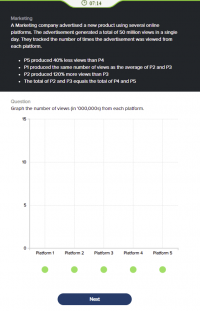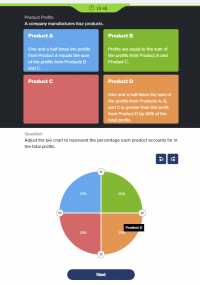Help on question from numerical reasoning test

bushra1175

Junior Member
I was hoping I could get some clues on how to work this out. I completely skipped the question because I didn't know where to begin. They didn't give me any starting values for any of the periods.

I only have one assumption. It says P2 & P3 equals P4 & P5. I would assume this means both sides equal 50%. I can't make sense of the other points. Some hints would be much appreciated.Harry_the_cat

Elite Member
You have 5 unknowns ie, the views on P1, P2, P3, P4 and P5.

For simplicity, I'd call them a,b,c,d,e, respectively.

To solve for 5 unknowns, you will need 5 equations.

Convert each statement into an equation.

"The ad generated a total of 50 million views in a single day" translates to a + b + c + d + e = 50 (million).

"P5 produced 40% less views than P4" translates to what?

Do the same for the other 3 statements.

Try to get 5 equations in total. Once you have 5 equations, you can solve them simultaneously. If you're not sure how, come back with your 5 equations and then we can guide you further.

BTW, your assumption is incorrect. If P2&P3 and P4&P5 are both 50%, that doesn't leave anything left for P1.

bushra1175

Junior Member
Sorry about that wrong assumption. It skipped my mind that there was also P1.

Here are my 5 equations:
P5 = P4 - (P4*0.4)
P1 = (P2+P3)/2
P2 = P3 + (P3*1.2)
P2 + P3 = P4 + P5

When you say we need to solve the equations simultaneously, do you mean like this?:

I feel like that would take long for a timed numerical reasoning test. I'm only allowed around 2 minutes per question sadly

Dr.Peterson

Elite Member
Sorry about that wrong assumption. It skipped my mind that there was also P1.

Here are my 5 equations:
P5 = P4 - (P4*0.4)
P1 = (P2+P3)/2
P2 = P3 + (P3*1.2)
P2 + P3 = P4 + P5

When you say we need to solve the equations simultaneously, do you mean like this?:

I feel like that would take long for a timed numerical reasoning test. I'm only allowed around 2 minutes per question sadly
There's one more equation (you only show 4), which comes from the opening paragraph.

It doesn't take too long to solve this by standard methods such as those introduced in your reference, once you have some experience with them; and there are some shortcuts you can take if you think rather than follow a routine method strictly. Give it a try. (I'd start with some substitutions to eliminate a couple variables immediately.)

I will add that I used variables a, b, c, d, e, which are easier to write and think about; and I took them to be in millions.

bushra1175

Junior Member
There's one more equation (you only show 4), which comes from the opening paragraph.

It doesn't take too long to solve this by standard methods such as those introduced in your reference, once you have some experience with them; and there are some shortcuts you can take if you think rather than follow a routine method strictly. Give it a try. (I'd start with some substitutions to eliminate a couple variables immediately.)

I will add that I used variables a, b, c, d, e, which are easier to write and think about; and I took them to be in millions.
I'll give it a go. I also would like to know if the process of simultaneous equations would need to be used for the below problem since there are many unknowns?Dr.Peterson

Elite Member
I'll give it a go. I also would like to know if the process of simultaneous equations would need to be used for the below problem since there are many unknowns?
View attachment 29569
Yes, this second problem can also be solved by a system of equations; and it's a little more work. There's an additional twist, in that there are only three equations for four unknowns. Fortunately, you are asked only for proportions; I would add in a fourth equation, A+B+C+D = 100, so that the four variables represent percentages of the total.

I don't see any better method.

•bushra1175